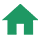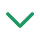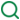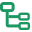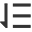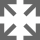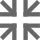### 一、类的私有变量

``````class Counter {
#x = 0;

#increment() {
this.#x++;
}

onClick() {
this.#increment();
}
}

const c = new Counter();
c.onClick(); // 正常
c.#increment(); // 报错
``````

### 二、可选链操作符

``````let nestedProp = obj && obj.first && obj.first.second;
``````

``````let nestedProp = obj?.first?.second;
``````

### 三、空位合并操作符

``````let c = a ? a : b // 方式1
let c = a || b // 方式2
``````

``````let c = a ?? b;
// 等价于let c = a !== undefined && a !== null ? a : b;
``````

``````const x = null;
const y = x ?? 500;
console.log(y); // 500
const n = 0
const m = n ?? 9000;
console.log(m) // 0
``````

### 四、BigInt

JS在Math上一直很糟糕的原因之一是，无法精确表示大于的数字2 ^ 53，这使得处理相当大的数字变得非常困难。

``````1234567890123456789 * 123;
// -> 151851850485185200000 // 计算结果丢失精度
``````

``````const aNumber = 111;
const aBigInt = BigInt(aNumber);
aBigInt === 111n // true
typeof aBigInt === 'bigint' // true
typeof 111 // "number"
typeof 111n // "bigint"
``````

``````1234567890123456789n * 123n;
// -> 151851850485185185047n
``````

``````1n < 2
// true

1n + 2
// Uncaught TypeError: Cannot mix BigInt and other types, use explicit conversions
``````

### 五、static 字段

``````class Colors {
// public static 字段
static red = '#ff0000';
static green = '#00ff00';

// private static 字段
static #secretColor = '#f0f0f0';

}

font.color = Colors.red;
font.color = Colors.#secretColor; // 出错
``````

### 六、Top-level await

ES2017（ES8）中的 async/await 特性仅仅允许在 async 函数内使用 await 关键字，新的提案旨在允许 await 关键字在顶层内容中的使用，例如可以简化动态模块加载的过程：

``````const strings = await import(`/i18n/\${navigator.language}`);
````````````// db.mjs
export const connection = await createConnection();
``````
``````// server.mjs
import { connection } from './db.mjs';
server.start();
``````

### 七、WeakRef

``````const ref = { x: 42, y: 51 };
// 只要我们访问 ref 对象（或者任何其他引用指向该对象），这个对象就不会被垃圾回收
``````

``````const wm = new WeakMap();
{
const ref = {};
wm.get(ref);
}
// 在这个块范围内，我们已经没有对 ref 对象的引用。
// 因此，虽然它是 wm 中的键，我们仍然可以访问，但是它能够被垃圾回收。

const ws = new WeakSet();
ws.has(ref);// 返回 true
``````

JavaScript 的 WeakMap 并不是真正意义上的弱引用：实际上，只要键仍然存活，它就强引用其内容。WeakMap 仅在键被垃圾回收之后，才弱引用它的内容。

WeakRef 是一个更高级的 API，它提供了真正的弱引用，Weakref 实例具有一个方法 deref，该方法返回被引用的原始对象，如果原始对象已被收集，则返回undefined对象。

``````const cache = new Map();
const setValue =  (key, obj) => {
cache.set(key, new WeakRef(obj));
};

const getValue = (key) => {
const ref = cache.get(key);
if (ref) {
return ref.deref();
}
};

// this will look for the value in the cache
// and recalculate if it's missing
const fibonacciCached = (number) => {
const cached = getValue(number);
if (cached) return cached;
const sum = calculateFibonacci(number);
setValue(number, sum);
return sum;
};
``````

## 参考文章